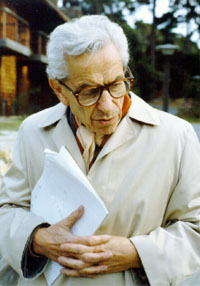# Breakthrough in Ramsey theory

### Breakthrough!

Progress in mathematics is often slow and difficult, and breakthroughs in even minor seeming problems can take years. Results in my field may require new tools, new inspiration, or new computational power to achieve. Sometimes it takes all of these to push the subject forward.

Last week, it was announced in a paper posted on arXiv that the fifth Ramsey number R(5)  is at most 48. Before this, it was known to be at most 49. To a random person, the response to this announcement would be a resounding “big deal”. But this is a breakthrough and I will explain why.

Have you ever looked up at the night sky and seen a pattern in the stars, one that doesn’t fit one of the known constellations? That is Ramsey theory at work, with your brain connecting the dots to find new patterns. What the math tells us is that patterns are truly unavoidable if there enough stars.What patterns do you see in the stars?

### Complete disorder is impossible

Ramsey theory is named after Frank P. Ramsey, who discovered a theorem in his 1928 paper On a problem of formal logic that led to an entire branch of mathematics. Ramsey worked in not only mathematics but also philosophy and economics. He died at the age of 26 after abdominal surgery.Frank P. Ramsey (1903-1930).

A classic example of Ramsey theory comes from so-called edge coloring graphs. In this setting, we consider a clique, or graph with all edges present between vertices. Now, color each edge red or blue; we call this a 2-coloring of the edges. The featured image of this blog is one such 2-coloring of a clique with 17 vertices. Note that if there are n vertices, then there about n2 many edges, so there are many ways to color the edges. For a clique with 49 vertices, there are 21176-many such colorings, which is much larger than the number of atoms in the universe. There are also a huge number of patterns by considering subgraphs with different colors.

One of the key insights of Ramsey theory is that no matter how you 2-color the edges, if the clique is large enough you will always find certain subgraphs with edges all of one color (or monochromatic). For example, if you 2-color the edges of a clique with 6 vertices, then there is always a red or blue triangle (with three vertices). A proof of this is as follows:

• Take a vertex x and consider its five neighbours.
• At least three edges incident with x must be one color, say red (or else we’d have at most four colored edges and there are 5 incident with x). Let the endpoints of these edges with x be a, b and c. See the figure below.
• If any of a, b and c have a red edge between them, then we have a red triangle (which includes x).
• If none of the edges between a, b, and c are red, then they form a blue triangle.This doesn’t happen if we use 5 or fewer vertices. Here is a 2-coloring of the clique with five vertices with no blue or red triangle.Based on this we say the Ramsey number R(3) = 6: in other words, the smallest clique so that any 2-coloring of the edges results in a monochromatic triangle has 6 vertices.

We can define R(n) for a general positive integer n in a similar way as R(3):  R(n) is the smallest integer m so that for any 2-coloring of the edges of the clique with m vertices, there is monochromatic clique with n vertices. Then R(n) defines a function with input a positive integer, and output the nth Ramsey number. It is straightforward to check that R(1)=1 and R(2) = 2. With more work, it can be shown that R(4) = 18.

### The aliens are coming

Wait… what is R(5)? No one knows. The best we knew (up until last week) was that R(5) is somewhere between 43 and 49. Paul Erdős told the famous story of aliens coming to earth and demanding to know the exact value of R(5) or they would destroy the Earth. Erdős’ advice was to have all the computers in the world work on the problem. If they came asking for R(6), then his advice was to leave the Earth.Paul Erdős (1913-1996).

It is humbling to think that we only know four exact values of R(n). There are infinitely many values of n, from 5 and up, where we don’t have a clue how to compute this function exactly.

### At most 48

Vigleik Angeltveit and Brendan McKay (both from Australian National University) posted a paper on arXiv in late March 2017 where they claim that 49 can be improved to 48. McKay and Radziszowski proved the previous best upper bound of 49 in 1997. So there was no progress on determining the value of R(5) for decades.

Their proof uses extensive computations, performing over 2 trillion graph operations they refer to as “gluing”. The extensive computing power needed would have been out of reach even a few years earlier.Brendan McKay, who is well-known for his work in computational graph theory.

While the paper needs verification through refereeing as it is posted as a preprint, this is a big development in Ramsey theory and likely will lead to new approaches towards discovering the true value of R(5).

An important take away for everyone reading this is that mathematics is mostly unfinished. Researchers are constantly discovering new mathematical truths, at least on the good days.

Anthony Bonato

## 2 thoughts on “Breakthrough in Ramsey theory”

1.Thomas

Impressive application, assuring the southern cross is hard to spot. R(5)!

Like